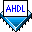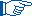## Using Constants & Evaluated Functions

 See AlsoYou can use a constant or evaluated function in an AHDL file to give a descriptive name to a number or text string (where the number or text string can be the value of an arithmetic expression). The descriptive name, which can be used in any section in an AHDL file, can be more informative than the number or text string. For example, the numeric constant `UPPER_LIMIT` can be more informative than the number `130`. Constants and evaluated functions can also be useful if the same number or text string is repeated several times in a file; if the number or text string changes, only one statement needs to be changed.You can use evaluated functions in arithmetic expressions only; you cannot use evaluated functions in Boolean expressions.

In AHDL, you implement constants with Constant Statements and evaluated functions with Define Statements. When you use a constant in an AHDL file, the Compiler replaces the constant with the number, text, or arithmetic expression value that is assigned to the constant in the Constant Statement. Similarly, when you use an evaluated function in an AHDL file, the Compiler replaces the evaluated function with the value of the arithmetic expression assigned to the evaluated function in the Define Statement (where the value is based on optional arguments for the expression).For the arithmetic expression that defines the constant or evaluated function, the Compiler evaluates each arithmetic operator and reduces the expression to a value (a number or text string). The Compiler does not generate logic for the expression. You can use previously defined constants, evaluated functions, or parameters to define constants and evaluated functions.  Example AHDL also provides the predefined evaluated functions `USED`, `CEIL`, and `FLOOR`.

The decode2.tdf file shown below has the same functionality as decode1.tdf (shown in Using Numbers), but uses the constant `IO_ADDRESS` instead of the number `H"0370"`.

```CONSTANT IO_ADDRESS = H"0370";

SUBDESIGN decode2
(
a[15..0] : INPUT;
ce       : OUTPUT;
)
BEGIN
END;
```

The strcmp.tdf file shown below defines the constant `FAMILY` and uses it in an Assert Statement to check whether the current device family is APEX 20K.

```PARAMETERS
(
DEVICE_FAMILY     % DEVICE_FAMILY is a predefined parameter %
);

CONSTANT FAMILY = "APEX20K";

SUBDESIGN strcmp
(
a : INPUT;
b : OUTPUT;
)
BEGIN
IF (DEVICE_FAMILY == FAMILY) GENERATE
ASSERT
REPORT "Detected compilation for APEX20K family"
SEVERITY INFO;
b = a;
ELSE GENERATE
ASSERT
REPORT "Detected compilation for % family"
DEVICE_FAMILY
SEVERITY ERROR;

b = a;
END GENERATE;
END;
```This example uses the predefined Altera® parameter `DEVICE_FAMILY`, which represents the target device family that you specified for the current design with the Device page of the Settings dialog box (Assignments menu).

The minport.tdf file shown below defines the evaluated function `EX`, which ensures a minimum port width in the Subdesign Section:

```PARAMETERS (WIDTH);

DEFINE EX(a,b) = (a > b) ? a : b;

SUBDESIGN minport
(
dataA[EX(WIDTH,0)..0] : INPUT;
dataB[EX(WIDTH,0)..0] : OUTPUT;
)
BEGIN
dataB[] = dataA[];
END;
```